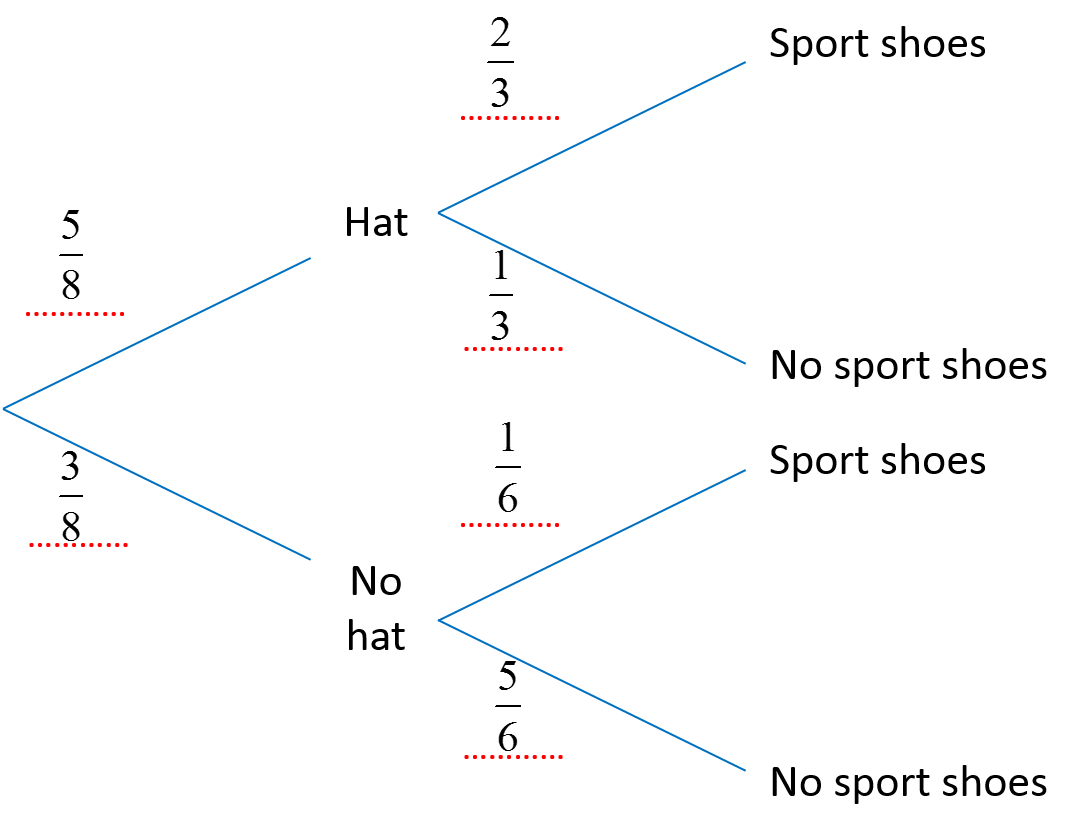# SPM Mathematics Trial 2021 (Selangor), Paper 2 (Question 7 & 8)

Question 7:
When Ivan goes to shopping mall, the probability that he wears a hat is 5/8. If he wears a hat, then the probability that he wears sport shoes is 2/3. If he does not wear a hat, then the probability that he wears sport shoes is 1/6.

(a) Diagram 4 in the answer space shows all the possible outcomes. Complete the tree diagram in Diagram 4. [2 marks](b) Hence, find the probability that Ivan wears a hat or sport shoes but not both. [2 marks]

Solution:
(a)(b)

Question 8:
Firdaus is training for a marathon. He practises according to his routine which is running as far as 32 km a week. He plans to increase his running distance, D(x), in km, each week by 10% of his running distance in the previous week, where x represents number of weeks of training.
Derive a mathematical model for his running distance, D(x) through mathematical modeling.
[4 marks]

Solution:

$10%=\frac{10}{100}=0.1$
D(x) = 32(1.1)x

 Week Calculation (km) Running distance (km) 1 32 + 32 × 0.1 = 32(1 + 0.1) 3.2 (1.1) 2 32(1.1) + 32(1.1) × 0.1 = 32(1.1)(1 + 0.1) 3.2 (1.1)2 3 32(1.1)2 + 32(1.1)2 × 0.1 = 32(1.1)2 (1 + 0.1) 3.2 (1.1)3 4 32(1.1)3 + 32(1.1)3 × 0.1 = 32(1.1)3 (1 + 0.1) 3.2 (1.1)4